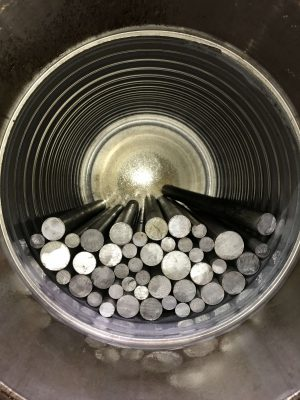# Rod milling in the Magotteaux MillGSL are currently running extensive flotation  trials on a base metal project, where the Magotteaux Mill is running a rod charge rather than a ball charge. This is done in order to better simulate the particle size distribution of a ball mill in closed circuit with a hydrocyclone in a continuous plant scenario.

The question arose what charge distribution to use, and especially what the void ratio between a graded charge of rods is.  After some trial and error it was decided to go for a rod charge of approximately 45kg. This equates to a charge of around 40% by volume, but this of course includes a certain void between rods. For a rod charge with a single diameter it is relatively well-documented what the packing and hence void ratio range can be expected to be. However, for a graded charge such as that used in this project, the packing becomes a much more complex matter. For instance, there are four smaller rods in the centre of the image which sit in a square arrangement that is far from optimal, and capturing this behaviour mathematically can be a very complicated process. Therefore, it was decided to use image processing to solve this question. Using Fiji, pictures of the rod charge were converted to black/white binary images, before segregating rod ends from the background and determining the area of the image occupied by rod ends. By comparing this to the charge level (percentage of the mill cylinder), it was possible to calculate that the rod charge in the picture equates to a void ratio of roughly 0.72. Though a somewhat academic exercise, it did highlight the value of image processing in mineral processing, and how straightforward it can be to exploit this potential. If you would like to discuss this further, you can contact us or go to the LinkedIn post.